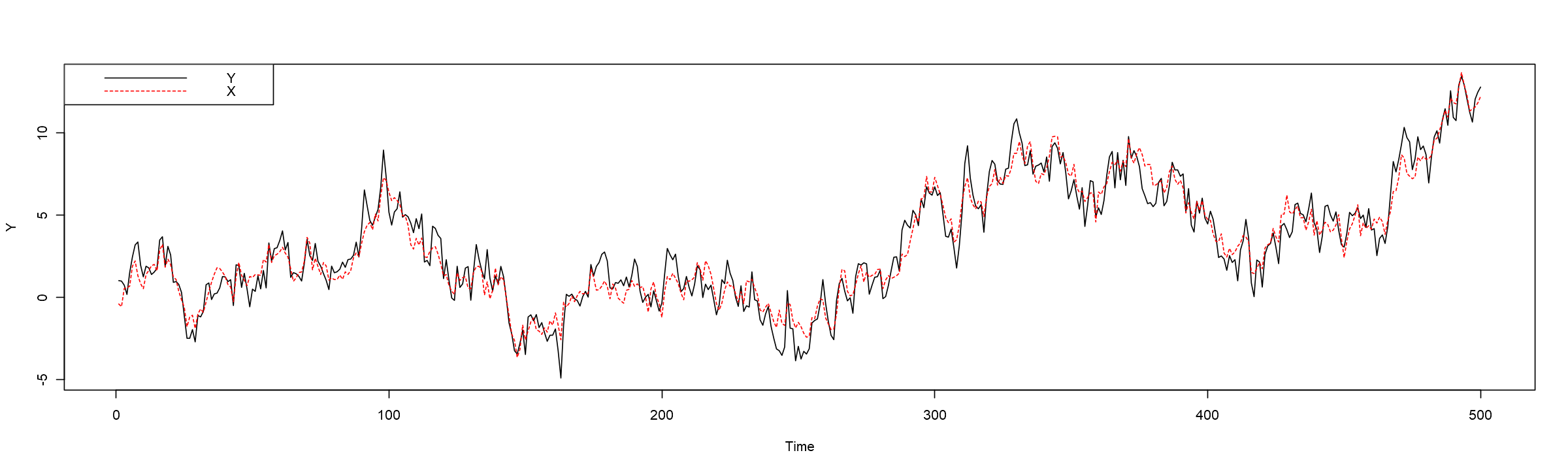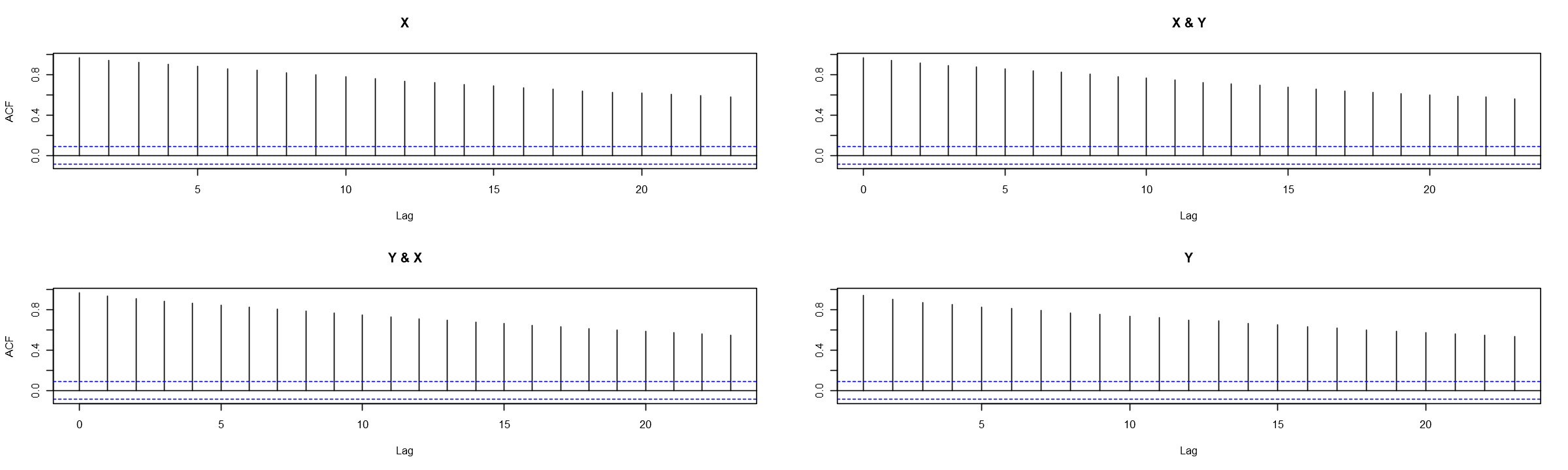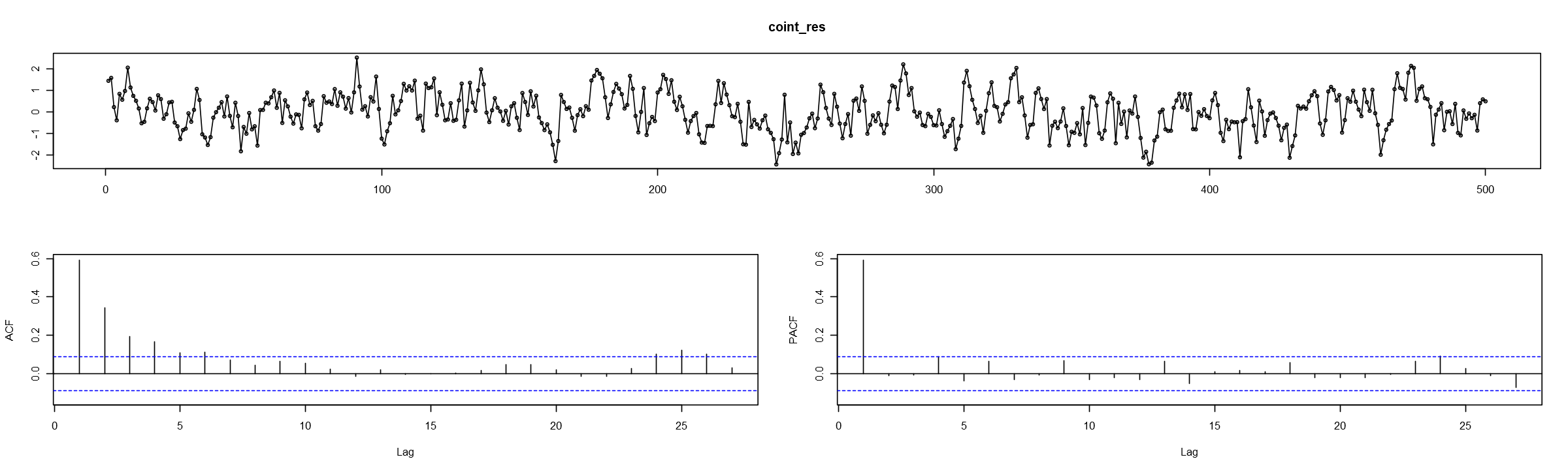In :
# Set plot options in Jupyter Lab
options(repr.plot.width = 20)
options(repr.plot.height = 6)


# Simulation example¶

Below we will simulate two cointegrated series.

\begin{aligned} Y_t &= \beta_2 X_t + u_t,\ u_t \sim I(0)\\ X_t &= X_{t-1} + v_t,\ v_t \sim I(0) \end{aligned}

where $\beta_2$ is the cointegrating parameter.

In this case $Y_t \sim I(1)$ and $X_t \sim I(0)$. Notice that the first equation gives the long-run equilibrium relationship $Y_t - \beta_2 X_t = u_t \sim I(0)$, i.e. the first equation gives the linear combination of $X_t$ and $Y_t$, which is stationary. The second relationship is the common stochastic trend (i.e. unit root) relationship that we are already familiar with.

Further examples can be found at this answer on stackoverflow. A more advanced example can be found in this paper.

For this example, we will choose:

• $\beta_2 = 1$ (notice that it does not matter what kind of parameter we choose, since the first equation will be equivalent to the cointegration relationship, as long as $u_t \sim I(0)$).
• $u_t = 0.6 u_{t-1} + \epsilon_t$, $\epsilon_t \sim \mathcal{N}(0, 0.5^2)$;
• $v_t \sim \mathcal{N}(0, 0.5^2)$;
In :
set.seed(123)
#
N <- 500
sd_u <- 0.5
sd_v <- 0.5
#
eps <- MASS::mvrnorm(N, mu = c(0, 0), Sigma = diag(c(sd_u, sd_v)))
#
u <- arima.sim(model = list(ar = 0.6), innov = eps[, 1], n = N)
v <- eps[, 2]
#
X <- cumsum(v)
Y <- X + u

In :
Y <- ts(Y)
X <- ts(X)


We can examine the series

In :
plot.ts(Y)
lines(ts(X), lty = 2, col = "red")
legend("topleft", legend = c("Y", "X"), lty = 1:2, col = c("black", "red"))We can examine their ACF and CCF plots:

In :
forecast::Acf(cbind(X, Y))We see that the series exhibit a slowly decaying ACF and a slowly decaying CCF.

• We can test the series for the precense of a unit root:
In :
tseries::adf.test(Y, alternative = "stationary")

	Augmented Dickey-Fuller Test

data:  Y
Dickey-Fuller = -2.2548, Lag order = 7, p-value = 0.4705
alternative hypothesis: stationary

In :
tseries::adf.test(X, alternative = "stationary")

	Augmented Dickey-Fuller Test

data:  X
Dickey-Fuller = -1.9203, Lag order = 7, p-value = 0.612
alternative hypothesis: stationary


In both cases, we do not reject the null hypothesis of a unit root.

• We next examine the series for coitegration:
In :
coint_reg <- lm(Y ~ X)

In :
coef(summary(coint_reg))

EstimateStd. Errort valuePr(>|t|)
(Intercept)-0.01864348 0.05471023 -0.3407676 7.334222e-01
X 1.00804449 0.01157911 87.0571908 1.872038e-303
In :
coint_res <- ts(coint_reg$res)  In : forecast::tsdisplay(coint_res)In : library(dynlm)  A nive feature of using R (or Python, or any other econometric software, which allows some form of coding) is that you can automate some of the boring procedures (only if you know that you can trust the automation process). In this case - we want to select the maximum lag for our residual unit root testing and reduce the maximum lag if it is insignificant - so, as this is a relatively trivial procedure, we can automate it: In : for(max_lag in 10:0){ print(paste0("Selecting max residual lag: ", max_lag)) if(max_lag > 0){ m_lag <- paste0("+ L(d(coint_res), 1:", max_lag, ")") }else{ m_lag <- "" } my_formula <- paste0("d(coint_res) ~ -1 + L(coint_res)", m_lag) my_formula <- as.formula(my_formula) resid_mdl <- dynlm(my_formula) if(tail(coef(summary(resid_mdl))[, 4], 1) < 0.05) break }   "Selecting max residual lag: 10"  "Selecting max residual lag: 9"  "Selecting max residual lag: 8"  "Selecting max residual lag: 7"  "Selecting max residual lag: 6"  "Selecting max residual lag: 5"  "Selecting max residual lag: 4"  "Selecting max residual lag: 3"  "Selecting max residual lag: 2"  "Selecting max residual lag: 1"  "Selecting max residual lag: 0"  In : round(coef(summary(resid_mdl)), 5)  EstimateStd. Errort valuePr(>|t|) L(coint_res)-0.40715 0.03596 -11.323240 The estimated residual model is:$ \Delta e_t = \rho e_{t-1} + w_t $Since our long-run equilibrium equation has one xogeneous variable,$X$, and no trend, the critical value is -3.37. The t-statistic of L(coint_res) is -11.32324. Since -11.32324 < -3.37, we reject the null hypothesis$H_0: \rho = 0$and conclude that the residuals do not have a unit root (this is to be expected, since we simulate$u_t$as stationary$\rm AR(1)$process. This also explains why the lagged values, L(d(coint_res)), we insignificant). This means that we reject the null hypothesis of no cointegration and conclude that$Y$and$X$are cointegrated. • We have found that$X_t, Y_t \sim I(1)$and$X_t$and$Y_t$are cointegrated - we now specify the$\rm ECM$: In : mdl_1 <- dynlm(d(Y) ~ 1 + L(coint_res) + L(d(Y), 1:3) + L(d(X), 0:3)) summary(mdl_1)  Time series regression with "ts" data: Start = 5, End = 500 Call: dynlm(formula = d(Y) ~ 1 + L(coint_res) + L(d(Y), 1:3) + L(d(X), 0:3)) Residuals: Min 1Q Median 3Q Max -2.03013 -0.46723 0.00755 0.47333 1.94879 Coefficients: Estimate Std. Error t value Pr(>|t|) (Intercept) -0.005645 0.032234 -0.175 0.8611 L(coint_res) -0.384014 0.048456 -7.925 1.57e-14 *** L(d(Y), 1:3)1 -0.024785 0.051707 -0.479 0.6319 L(d(Y), 1:3)2 -0.024013 0.048265 -0.498 0.6190 L(d(Y), 1:3)3 -0.083953 0.044979 -1.866 0.0626 . L(d(X), 0:3)0 1.060436 0.046979 22.573 < 2e-16 *** L(d(X), 0:3)1 0.074160 0.070645 1.050 0.2943 L(d(X), 0:3)2 0.080390 0.067945 1.183 0.2373 L(d(X), 0:3)3 0.100581 0.065631 1.533 0.1260 --- Signif. codes: 0 '***' 0.001 '**' 0.01 '*' 0.05 '.' 0.1 ' ' 1 Residual standard error: 0.7158 on 487 degrees of freedom Multiple R-squared: 0.568, Adjusted R-squared: 0.5609 F-statistic: 80.04 on 8 and 487 DF, p-value: < 2.2e-16  Again, we see insignificant variables. No that this can also be automated and you should try to do this following the previous example. We instead move to the finalized model: In : mdl_1 <- dynlm(d(Y) ~ 1 + L(coint_res, 1) + L(d(X), 0)) summary(mdl_1)  Time series regression with "ts" data: Start = 2, End = 500 Call: dynlm(formula = d(Y) ~ 1 + L(coint_res, 1) + L(d(X), 0)) Residuals: Min 1Q Median 3Q Max -1.94632 -0.45223 -0.01014 0.47760 1.95487 Coefficients: Estimate Std. Error t value Pr(>|t|) (Intercept) -0.003525 0.032058 -0.11 0.912 L(coint_res, 1) -0.407843 0.035997 -11.33 <2e-16 *** L(d(X), 0) 1.056106 0.046600 22.66 <2e-16 *** --- Signif. codes: 0 '***' 0.001 '**' 0.01 '*' 0.05 '.' 0.1 ' ' 1 Residual standard error: 0.7156 on 496 degrees of freedom Multiple R-squared: 0.5606, Adjusted R-squared: 0.5588 F-statistic: 316.3 on 2 and 496 DF, p-value: < 2.2e-16  Note that L(d(X), 0) is equivalent to d(X). The estimated model is:$\Delta Y_t = -0.003525 - 0.407843 \widehat{e}_{t-1} + 1.056106 \Delta X_t + \epsilon_t$. where: • The short-run multiplier of$X$is 1.056106; • The long-run multiplier of$X$is 1.00804449, which we get from the cointegration regression: In : coef(summary(coint_reg))  EstimateStd. Errort valuePr(>|t|) (Intercept)-0.01864348 0.05471023 -0.3407676 7.334222e-01 X 1.00804449 0.01157911 87.0571908 1.872038e-303 ## How does the ECM relate to our true model?¶ Remember that we have simulated the following model:$ \begin{cases} Y_t &= \beta_2 X_t + u_t,\\ u_t &= \widetilde{\phi} u_{t-1} + \epsilon_t, \epsilon_t \sim I(0)\\ X_t &= X_{t-1} + v_t,\ v_t \sim I(0) \end{cases} $As we have done before, we can express$u_t = Y_t - \beta_2 X_t$to get$Y_t - \beta_2 X_t = \widetilde{\phi} Y_{t-1} - \widetilde{\phi} \beta_2 X_{t-1} + \epsilon_t$. Finally, our re-written model is:$ Y_t = \widetilde{\phi} Y_{t-1} + \beta_2 X_t - \widetilde{\phi} \beta_2 X_{t-1} + \epsilon_t $Looking at the lecture slides, we see that we can re-write the model as the ECM:$ \Delta Y_t = \beta_0 \Delta X_t - (1 - \widetilde{\phi}) \left[ Y_{t-1} - \widetilde{\beta} X_{t-1} \right] + \epsilon_t $where: •$\beta_0 = \beta_2 = 1$; •$- (1 - \widetilde{\phi}) = -0.4$; •$\widetilde{\beta} = \beta_2 = 1$; If we compare the true values with the estimated ones from the model output - we see that they are the same (our model also includes the intercept component, however, it is insignificant). ## Relationship between$X_t$,$Y_t$,$u_t$and ECM¶ Note that in the above expression we got that$1 - \widetilde{\phi} \neq 0$, since$\widetilde{\phi}$is the autoregressive parameter of$u_t$(and NOT for$Y_t$). However, this does not mean that$Y_t$is stationary. As mentioned before, both$X_t$and$Y_t$are$I(1)$. To see why, rewrite$X_t = 1/\beta_2 (Y_t - u_t)$and insert it into the expression of$X_t$, to get$1/\beta_2 (Y_t - u_t) = 1/\beta_2 (Y_{t-1} - u_{t-1}) + v_t$, which we can write as:$ Y_t = Y_{t-1} + \Delta u_t + \beta_2 v_t $In other words,$Y_t \sim I(1)$. However the above expression contains two different random components$u_t$and$v_t$, which we cannot evaluate separately. Hence why the previous expression only highlights the fact that$Y_t ~ \sim I(1)\$, while the ECM form and the long-run equilibrium regression allows us to estimate all the relevant parameters.

In [ ]:


In [ ]: